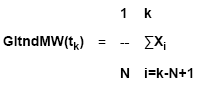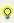# Floating average

The moving average is re-calculated and displayed after each change. The first output of the value occurs when all changes are present.

Press the button OK to open a dialog in which you can enter the number of values to be considered for the calculation:

Formula

Xi = Measured value of the source variable

ti = Time of the Xi measured value

T = Time interval of the averaging (query after confirming the definition of the formula)

N = Number of the received measured values within the interval T

k = current timeInfo The calculation is intended for filtering out small fast oscillations. The algorithm expects that the source values arrive regularly in approximately equal steps (smallest step between two events is 500 ms), so that the calculation can be performed in event-triggered mode (fixed scan rate). That means: the calculation is not really suitable for spontaneous drivers.